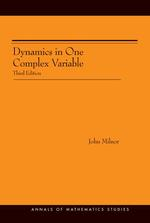# Dynamics in One Complex Variable. (AM-160): Third Edition. (AM-160)

John Milnor
https://doi.org/10.2307/j.ctt7rnxn
Pages: 310
https://www.jstor.org/stable/j.ctt7rnxn

1. Front Matter
(pp. i-iv)
(pp. v-v)
3. LIST OF FIGURES
(pp. vi-vi)
4. PREFACE TO THE THIRD EDITION
(pp. vii-vii)
John Milnor
5. CHRONOLOGICAL TABLE
(pp. viii-viii)
6. RIEMANN SURFACES
(pp. 1-38)

The first three sections will present an overview of some background material.

If$V\; \subset \;\mathbb{C}$is an open set of complex numbers, a function$f:\;V\; \to \;\mathbb{C}$is calledholomorphic(or “complex analytic”) if the first derivative

$z \mapsto f'(z)\; = \;\mathop {{\text{lim}}}\limits_{h \to 0} \,(f(z + h)\; - \;f(z))\,/\,h$

is defined and continuous as a function from$V$to$\mathbb{C}$, or equivalently if$f$has a power series expansion about any point${z_0}\; \in \;V$which converges to$f$in some neighborhood of${z_0}$. (See, for example, Ahlfors .) Such a function isconformalif the derivative$f'(z)$never vanishes. Thus our conformal maps must always preserve orientation. It isunivalent(orschlicht) if it is...

7. ITERATED HOLOMORPHIC MAPS
(pp. 39-75)

The study of iterated holomorphic mappings began in the 19th century but only came to flower in the 20th. One primary focus in the 19th century was the study of functional equations relating different known or unknown functions. Charles Babbage , “An essay on the calculus of functions,” set the stage with an attempt to understand many such functional equations. In particular (on page 412), he implicitly described what we now call asemiconjugacy$\varphi$between two different functions$f$and$g$, that is a map satisfying$\varphi \circ f = g \circ \varphi$so that the following diagram is commutative:

$\begin{array}{*{20}{c}} X & {\xrightarrow{f}} & X \\ {\varphi \downarrow } & {} & {\varphi \downarrow } \\ Y & {\xrightarrow{g}} & {Y.} \\ \end{array}$.

It follows that any...

8. LOCAL FIXED POINT THEORY
(pp. 76-141)

The next four sections will study the dynamics of a holomorphic map in some small neighborhood of a fixed point. This local theory is a fundamental tool in understanding more global dynamics. It has been studied for well over a hundred years by mathematicians such as Ernst Schröder, Gabriel Kœnigs, Léopold Leau, Lucjan Böttcher, Pierre Fatou, Gaston Julia, Hubert Cremer, Carl Ludwig Siegel, Thomas Cherry, Alexander Bryuno, Jean Écalle, Serguei Voronin, Michel Herman, Jean-Christophe Yoccoz, and Ricardo Perez-Marco. In most cases it is now well understood, but a few cases still present extremely difficult problems.

We start by expressing our...

9. PERIODIC POINTS: GLOBAL THEORY
(pp. 142-160)

The number of fixed points of a rational map$f:\;\hat \mathbb{C} \to \hat \mathbb{C}$of degree$d\; \ge \;0,$can be counted as follows.

Lemma 12.1.If$f$is not the identity map, then$f$has exactly$d + 1$fixed points, counted with multiplicity.

Here themultiplicityof a finite fixed point$\hat z\; = \;f(\hat z)$is defined to be the unique integer$m\; \ge \;1$for which the power series expansion of$f(z)\; - \;z$about${\hat z}$has the form

$f(z)\; - \;z\; = \;{a_m}{(z - \hat z)^m} + {a_{m + 1}}{(z - \hat z)^{m + 1}} + \; \cdots$

with${a_m} \ne 0$. Thus$m \ge 2$if and only if the multiplier$\lambda$at${\hat z}$is exactly 1. (Note that${\hat z}$is then a parabolic point with$m - 1$attracting petals, each of which maps...

10. STRUCTURE OF THE FATOU SET
(pp. 161-173)

This section will be a survey, without complete proofs, describing a close relative of the Siegel disk.

Definition. A componentUof the Fatou set$\hat \mathbb{C}\,J(f)$is called aHerman ringifUis conformally isomorphic to some annulus

${\mathcal{A}_r}\; = \;\{ z\,;\;1\; < \;|\,z\,|\; < \;r\}$,

and iff, or some iterate off, corresponds to an irrational rotation of this annulus. (Siegel disks and Herman rings are often collectively calledrotation domains.)

There are two known methods for constructing Herman rings. The original method, due to Herman , is based on a careful analysis of real analytic diffeomorphisms of the circle. An alternative method, due to...

11. USING THE FATOU SET TO STUDY THE JULIA SET
(pp. 174-218)

Carathéodory’s theory of “prime ends” is the basic tool for relating an open set of complex numbers to its complementary closed set. LetUbe a simply connected subset of$\hat \mathbb{C}$such that the complement$\hat \mathbb{C}U$is infinite. The Riemann Mapping Theorem asserts that there exists a conformal isomorphism

$\psi :\;\mathbb{D}\;\xrightarrow{ \cong }\;U$.

In some cases,$\psi$will extend to a homeomorphism from the closed disk${\bar \mathbb{D}}$onto the closure$\bar U,$, so that the topological boundary$\partial U$is homeomorphic to the circle$\partial \mathbb{D}$. (See Figures 5a (p. 42) and 37a, together with Theorem 17.16.) However, this is not true in general, since the...

12. Appendix A. Theorems from Classical Analysis
(pp. 219-225)
13. Appendix B. Length-Area-Modulus Inequalities
(pp. 226-233)
14. Appendix C. Rotations, Continued Fractions, and Rational Approximation
(pp. 234-245)
15. Appendix D. Two or More Complex Variables
(pp. 246-253)
16. Appendix E. Branched Coverings and Orbifolds
(pp. 254-258)
17. Appendix F. No Wandering Fatou Components
(pp. 259-265)
18. Appendix G. Parameter Spaces
(pp. 266-270)
19. Appendix H. Computer Graphics and Effective Computation
(pp. 271-276)
20. REFERENCES
(pp. 277-292)
21. INDEX
(pp. 293-304)